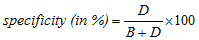# true negative

Also found in: Dictionary, Thesaurus, Legal, Acronyms, Encyclopedia.
Related to true negative: False Negative Rate

## true negative

Statistics A negative test result, that accurately reflects the tested-for activity of an analyte

## specificity

The extent to which a test gives results that are free from false positives (i.e. people found to have the defect when they are actually free of it). The fewer the number of false positives, the greater is the specificity of the test. It is usually presented as the percentage of people truly identified as not defectives, or normal, referred to as true negatives, D (or correct reject), divided by the total number of not defectives or normal people tested. The total number includes all the true negatives, D, plus the false positives, B (or false alarm). HenceSee sensitivity.
References in periodicals archive ?
This gives 16 true positive, 6 false positive, 11 false negative and 17 true negative cases.
Out of 115 cases included in the present study 73 cases were available for histopathological correlation, 59 cases were true positive, 11 cases were true negative, one case was false positive and two cases were false negative.
To assess the performance of these methods, 6 criteria were used: True positive rate (TP) or CTS detection rate; False positive rate (FP) or false alarm ratio; True negative rate (TN); False negative rate (FN); Accuracy (AC); and Preciseness (PR).
The true negatives are 15 and false negatives are 5 with a sensitivity of the test being 94.
Out of 56 cases of clinically diagnosed potentially malignant lesions, VELscope detected overall 39 cases as true positive, 4 cases as true negative, 1 case as false positive, and 12 cases as false negative with sensitivity of 76.
If we consider an example similar to the above, and run our assay on 50 true negative samples with results calling 48 of the 50 negative, we have a specificity of 96 percent.
True positive, true negative, false positive, and false negative results of MSCT in the group with the middle and proximal segments of the coronary arteries and more than 50% stenosis Groups TPS TNS FPS FNS Middle and proximal segments more than 50% stenosis 127 349 4 4 TPS: true positive segments; TNS: true negative segments; FPS: false positive segments; FNS: false negative segments Table 2.
This means that the predictors had relatively good ability to identity true negative cases, that is, patients with low risk.
The accuracy was defined as dividing the true positive and true negative cases by all the cases studied.
This discriminative potential can be quantified by the measures of diagnostic accuracy such as true positive rate (sensitivity), true negative rate (specificity), positive predictive value (PPV), negative predictive value (NPV), likelihood ratio positive (LR+), likelihood ratio negative (LR-), area under the ROC curve, Youden's index, and diagnostic odds ratio (DOR).

Site: Follow: Share:
Open / Close